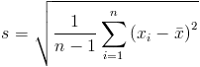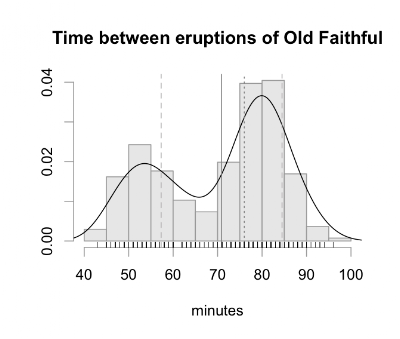## Monday, April 26, 2010

### Using R for Introductory Statistics, Chapters 1 and 2

I'm working my way through Using R for Introductory Statistics, by John Verzani, a free version of which is available as SimpleR.

#### Chapter 1

...covers basics of R such as arithmetic, loading libraries and reading data. We also get an introduction to vectors and indexing.

#### Chapter 2: Univariate Data

The book divides data into three types: categorical, discrete numerical and continuous numerical. Other books talk about levels or scales of measurement: nominal (same as categorical), ordinal (rank), interval (arbitrary zero), and ratio (true zero).

The table command tabulates categorical observations.

```> table(central.park.cloud)
central.park.cloud
clear partly.cloudy        cloudy
11            11             9
```

We can use cut to bin numeric data.

```> attach(faithful)
> bins = seq(42,109,by=10)
> freqs <- table(cut(waiting,bins))
```

For summarizing a data series, use the summary command, or its cousin fivenum. Fivenum gives the Tukey five number summary (minimum, lower-hinge, median, upper-hinge, maximum). Hinges are the medians of the left and right halves of the data, which is only slightly different than quartiles.

```> summary(waiting)
Min. 1st Qu.  Median    Mean 3rd Qu.    Max.
43.0    58.0    76.0    70.9    82.0    96.0
```

The two most common measures of central tendency are mean and median. Variance and standard deviation measure how much variation there is from the mean. They are measures of dispersion or spread.The standard deviation, the square root of the variance, has the same units as the original data.I've personally always wondered why we square the differences rather than take the distance or mean absolute deviation. Apparently, it's a matter of some debate.

Other measures of variability or dispersion are quantiles (quantile) and inter-quartile range (IQR).

Histograms are a graphical way to look at how data points are distributed over a range. To construct a histogram, we first divide the data into bins. Then, for each bin, we draw a rectangle whose area is proportional to the frequency of data that falls into that bin. Drawing histograms in R is done with the hist command.

```par(fg=rgb(0.6,0.6,0.6))
hist(waiting, breaks='scott', prob=T,
col=rgb(0.9,0.9,0.9),
main='Time between eruptions of Old Faithful',
ylab=NULL, xlab='minutes')
par(fg='black')
lines(density(waiting))
abline(v=mean(waiting), col=rgb(0.5,0.5,0.5))
abline(v=median(waiting), lty=3, col=rgb(0.5,0.5,0.5))
abline(v=mean(waiting)+sd(waiting), lty=2, col=rgb(0.7,0.7,0.7))
abline(v=mean(waiting)-sd(waiting), lty=2, col=rgb(0.7,0.7,0.7))
rug(waiting)
```Boxplots give another way of viewing the shape of data which works for comparing several distributions, although this example shows only one.

```library(UsingR)
attach(alltime.movies)
f = fivenum(Gross)
boxplot(Gross, ylab='all-time gross sales', col=rgb(0.8,0.8,0.8))
text(rep(1.35,5), f, labels=c('minimum', 'lower hinge', 'median', 'upper hinge', 'maximum'), cex=0.6)
```